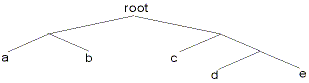# Huffman Compression

Background:

When we encode characters in computers, we assign each an 8-bit code based on an ASCII chart. But in most files, some characters appear more often than others. So wouldn't it make more sense to assign shorter codes for characters that appear more often and longer codes for characters that appear less often?

This is exactly what Claude Shannon and R.M. Fano were thinking when they created the first compression algorithm in the 1950's. However, D.A. Huffman published a paper in 1952 that improved the algorithm slightly and it soon superceded Shannon-Fano coding with the appropriately named Huffman coding.

The Concept:

Huffman coding has the following properties:

• Codes for more probable characters are shorter than ones for less probable characters.
• Each code can be uniquely decoded
• To accomplish this, Huffman coding creates what is called a "Huffman tree", which is a binary tree such as this one:Figure: a sample Huffman tree

To read the codes from a Huffman tree, start from the root and add a '0' every time you go left to a child, and add a '1' every time you go right. So in this example, the code for the character 'b' is 01 and the code for 'd' is 110.

As you can see, 'a' has a shorter code than 'd'. Notice that since all the characters are at the leafs of the tree (the ends), there is never a chance that one code will be the prefix of another one (eg. 'a' is 01 and 'b' is 011). Hence, this unique prefix property assures that each code can be uniquely decoded.

The Algorithm:

So how is this wonderful, magical Huffman tree created, you ask? Here is the algorithm, as taken from "The Data Compression Book":

First count the amount of times each character appears, and assign this as a "weight" to each character, or node. Add all the nodes to a LIST.

Then, repeat these steps until there is only one node left:

• Find the two nodes with the lowest weights.
• Create a parent node for these two nodes. Give this parent node a weight of the sum of the two nodes.
• Remove the two nodes from the list, and add the parent node.
• This way, the nodes with the highest weight will be near the top of the tree, and have shorter codes. Try the algorithm on the following characters:

a: 7
b: 6
c: 5
d: 2
e: 1

You should get a tree similar to the one above.

From this point, you simply get the codes for each character and send the encoded string along with the tree. So the string 'abc' will be 000110.

The decoder then can use the Huffman tree to decode the string by following the paths according to the string and adding a character every time it comes to one.

The Applet:

This is an applet written by Walter Korman for an excellent article on compression "Data Compression: Bits, Bytes and Beefalo" in Deep Magic. I modified it slightly so that it can accept a string from a form, but all the credit goes to him.

All you have to do is enter a string into the form and press "Run Applet". The applet demonstrates the encoding and decoding of the string, but not the building of the tree.

Try strings with only a few characters that are repeated different amounts.
For example: "aaabbccddddddeee" or "yabba dabba doo".

 String to Encode:

If you would like the Java source code for the applet:
Huffman.java and BaseApplet.java.